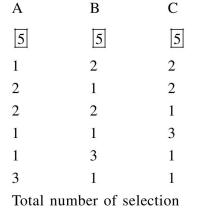# There are 3 sections in a question paper and each section contains 5 questions.

Question:

There are 3 sections in a question paper and each section contains 5 questions. A candidate has to answer a total of 5 questions, choosing at least one

question from each section. Then the number of ways, in which the candidate can choose the questions, is:

1. 1500

2. 2255

3. 3000

4. 2250

Correct Option: , 4

Solution:$=\left({ }^{5} \mathrm{C}_{1}{ }^{5} \mathrm{C}_{2}{ }^{5} \mathrm{C}_{2}\right) \cdot 3+\left({ }^{5} \mathrm{C}_{1}{ }^{5} \mathrm{C}_{1}{ }^{5} \mathrm{C}_{3}\right) \cdot 3$

$=5 \cdot 10 \cdot 10 \cdot 3+5 \cdot 5 \cdot 10 \cdot 3$

$=2250$